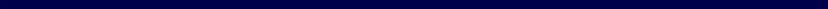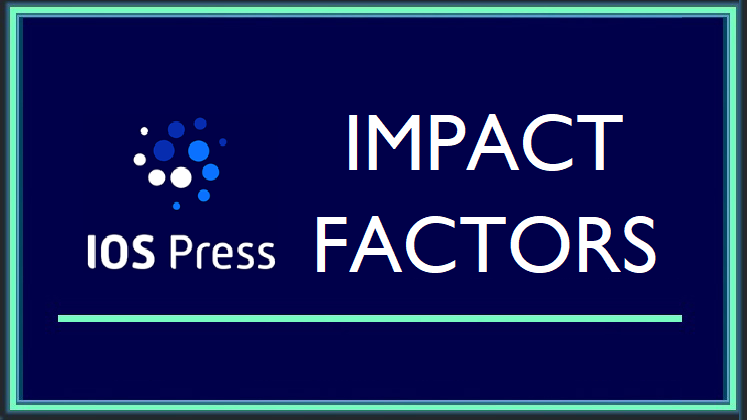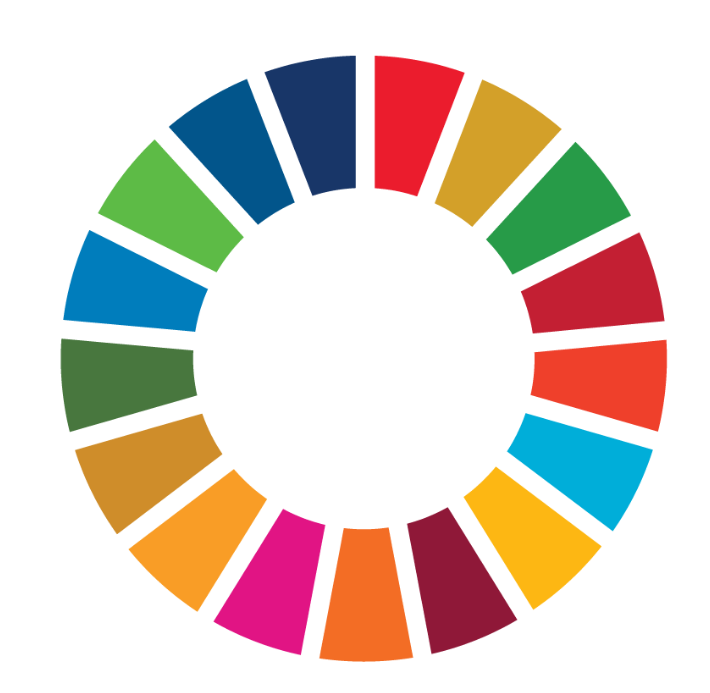Like   Tweet   in
 /* styles */ Discover IOS Press' COVID-19 content and latest news | IOS Press Quarterly Update | Dispatch Date: Jul 22, 2022
 /* styles */  ‌ ‌ ‌ ‌ ‌ ‌ ‌ ‌ ‌ ‌ ‌ ‌ ‌ ‌ ‌ ‌ ‌ ‌ ‌ ‌ ‌ ‌ ‌ ‌ ‌ ‌  In the News | Journals | Books | Tech | COVID-19 | Open Access | Share# IOS Press News

During 2022 Quarter 2 (Q2, Apr–Jun), we have been busy working on a number of initiatives and innovations behind the scenes, some of which are shared here.

 table div table+table+table+table+table+table div table{width:100%;padding:0}table div table+table+table+table+table+table div table img{width:96.23%;padding:0;float:none}table div table+table+table+table+table+table div table td{width:100%;padding:0 1.88% 18px}/* styles */## GO FAIR Foundation

We are pleased to share that we have been furthering our partnership with GO FAIR Foundation in Q2 2022. We advocate for transparency when it comes to data. The implementation of guiding principles to make data findable, accessible, interoperable, and reusable (FAIR) relies on public endorsement and cooperation, and we are pleased to collaborate with GO FAIR as we strive to make content as accessible as possible in line with these guiding principles. Researchers and the wider data communities are advancing open science culture through Implementation Networks, which are core drivers of the GO FAIR initiative as vehicles for advancing the global discussion about data stewardship. We continually work on advancing FAIR data and you can view related articles via the links below.

 table div table+table+table+table+table+table+table+table div table,table.module-7{width:49.62%;float:left;padding:0}table div table+table+table+table+table+table+table+table div table a{border:0 none;text-decoration:none}table div table+table+table+table+table+table+table+table div table img{width:100%!important;border:0 none;text-decoration:none}table div table+table+table+table+table+table+table+table div table td{width:100%;padding:0 20px 20px 0}/* styles */ Coming Soon: StemData FAIR data will be high on the agenda in the upcoming launch of StemData to publish research on the computational biology of stem cells, which will be a component of the new StemHub (see an update on this below). Read More
 table div table+table+table+table+table+table+table+table+table div table,table.module-8{width:49.43%;float:left;padding:0}table div table+table+table+table+table+table+table+table+table div table a{border:0 none;text-decoration:none}table div table+table+table+table+table+table+table+table+table div table img{width:100%!important;border:0 none;text-decoration:none}table div table+table+table+table+table+table+table+table+table div table td{width:100%;padding:0 20px 20px 0}/* styles */ GO FAIR Implementation Network The implementation of guiding principles to make data findable, accessible, interoperable, and reusable (FAIR) relies on public endorsement and cooperation. This Labs post highlighted the objectives for a proposed new network. Read More
 table div table+table+table+table+table+table+table+table+table+table div table,table.module-9{width:49.81%;float:left;padding:0}table div table+table+table+table+table+table+table+table+table+table div table a{border:0 none;text-decoration:none}table div table+table+table+table+table+table+table+table+table+table div table img{width:100%!important;border:0 none;text-decoration:none}table div table+table+table+table+table+table+table+table+table+table div table td{width:100%;padding:0 20px 20px 0}/* styles */ FAIR Principles for Research Software A special issue of Data Science – on FAIR data – covered analysis of the principles directly applied to software. This Labs post introduced the four foundational FAIR principles in the context of research data. Read More
 table div table+table+table+table+table+table+table+table+table+table+table div table{width:100%;padding:0}table div table+table+table+table+table+table+table+table+table+table+table div table img{width:96.23%;padding:0;float:none}table div table+table+table+table+table+table+table+table+table+table+table div table td{width:100%;padding:0 1.88% 18px}/* styles */## DataLENS

We are pleased to announce support of the DataLENS initiative, which provides omics analysis and visualization that will be of interest and tailored specifically for Alzheimer’s disease (AD) researchers. The aim of this open data portal is to advance AD research by making data available to all neuroscientists. The project was established by the Massachusetts Center for Alzheimer Therapeutics Science, and explained during the IOS Press symposium contribution of Sudeshna Das, assistant professor of neurology at Massachusetts General Hospital and Harvard Medical School. IOS Press is now supporting the ongoing development of the DataLENS 2.0 platform. View DataLENS Presentation

 /* styles */ Look out for an upcoming post on DataLENS by Sudeshna Das that will be published soon on the Labs website here!
 table div table+table+table+table+table+table+table+table+table+table+table+table+table+table div table{width:100%;padding:0}table div table+table+table+table+table+table+table+table+table+table+table+table+table+table div table img{width:96.23%;padding:0;float:none}table div table+table+table+table+table+table+table+table+table+table+table+table+table+table div table td{width:100%;padding:0 1.88% 18px}/* styles */## Hybrid Intelligence

Frank van Harmelen, director of the Hybrid Intelligence Centre at the Vrije Universiteit Amsterdam, gave an interesting talk about machines as scientific colleagues at the IOS Press symposium recently. Following on from this, we were pleased to be a sponsor of the very first international conference on Hybrid Human-Artificial Intelligence that took place in Leiden in June 2022. The conference proceedings will be published in the IOS Press book series Frontiers in Artificial Intelligence and Applications (FAIA) in due course, and to be kept updated you can sign up for news. Get FAIA Updates

 /* styles */ You can read about the IOS Press 35th anniversary in the report on Labs, and be sure to check out the online contributions via this page!# Journals

IMPACT FACTORS: The latest Journal Citation Reports (Source: Clarivate, 2022) was released in June 2022 and we are pleased to announce the continued growth of Impact Factors for our journals.Overall, IOS Press has a 15% increase in the Impact Factors across its 29 titles currently covered in the Journal Citation Reports, with a notable first impact factor announcement for one of our Medicine & Health journals. The Journal of Neuromuscular Diseases is in the Neurosciences category of the Web of Science and it has an Impact Factor of 4.693.

IOS Press Journals with Increased Impact Factors*

Integrated Computer-Aided Engineering Impact Factor 6.137
Journal of Neuromuscular Diseases Impact Factor 4.693 (view JND announcement)
Semantic Web Impact Factor 3.105
Restorative Neurology and Neuroscience Impact Factor 2.976
Journal of Ambient Intelligence and Smart Environments Impact Factor 2.759
Journal of X-Ray Science and Technology Impact Factor 2.442
Clinical Hemorheology and Microcirculation Impact Factor 2.411
WORK Impact Factor 1.803
Journal of Back and Muskuloskeletal Rehabilitation Impact Factor 1.456
Applied Ontology Impact Factor 1.367
Intelligent Data Analysis Impact Factor 1.321
Asymptotic Analysis Impact Factor 1.259
AI Communications Impact Factor 1.029
Isokinetics and Exercise Science Impact Factor 0.729

*Journal Citation Reports (Source: Clarivate, 2022)

 table div table+table+table+table+table+table+table+table+table+table+table+table+table+table+table+table+table+table+table+table div table{width:100%;padding:0}table div table+table+table+table+table+table+table+table+table+table+table+table+table+table+table+table+table+table+table+table div table img{width:96.23%;padding:0;float:none}table div table+table+table+table+table+table+table+table+table+table+table+table+table+table+table+table+table+table+table+table div table td{width:100%;padding:0 1.88% 18px}/* styles */## JOURNAL NEWS

 table div table+table+table+table+table+table+table+table+table+table+table+table+table+table+table+table+table+table+table+table+table+table div table,table.module-21{width:20.19%;float:right;padding:0}table div table+table+table+table+table+table+table+table+table+table+table+table+table+table+table+table+table+table+table+table+table+table div table a{border:0 none;text-decoration:none}table div table+table+table+table+table+table+table+table+table+table+table+table+table+table+table+table+table+table+table+table+table+table div table img{width:100%!important;border:0 none;text-decoration:none}table div table+table+table+table+table+table+table+table+table+table+table+table+table+table+table+table+table+table+table+table+table+table div table td{width:100%;padding:0 0 20px 20px}/* styles */ Advances in Communication and Swallowing | The first issue of 2022 (Vol.25, Iss.1), published in June 2022, includes the journal's first COVID-19 article, the spotlight paper covering speech and language therapy, and much more. A press release for an ACS paper was also issued in Q2, 2022, and the journal's first spring lecture took place in April, which was presented by lead author Shawn O'Keefe, MD (National University of Ireland, Galway) and covered the award-winning review article (as selected by the Editorial Board), which is the top viewed ACS paper since its launch in 2021. View Issue | Read Press Release | Watch Lecture Recording
 table div table+table+table+table+table+table+table+table+table+table+table+table+table+table+table+table+table+table+table+table+table+table+table div table,table.module-22{width:20.19%;float:right;padding:0}table div table+table+table+table+table+table+table+table+table+table+table+table+table+table+table+table+table+table+table+table+table+table+table div table a{border:0 none;text-decoration:none}table div table+table+table+table+table+table+table+table+table+table+table+table+table+table+table+table+table+table+table+table+table+table+table div table img{width:100%!important;border:0 none;text-decoration:none}table div table+table+table+table+table+table+table+table+table+table+table+table+table+table+table+table+table+table+table+table+table+table+table div table td{width:100%;padding:0 0 20px 20px}/* styles */ Information Polity | A call for papers has been issued on “Digital Government and Sustainable Development Goals." The guest editors, Rony Medaglia and Gianluca Misuraca, seek contributions on digital strategies in relation to the UN SDGs at global, national, and local level: "We welcome research from different social science perspectives, including Public Administration, Information Systems, Sociology, Information Science, and Management, that can combine relevant research foci with rigorous methodological approaches. Interdisciplinary submissions are also encouraged, as well as submissions that are theoretical and/or empirically based." View Call For Papers | Visit Journal Website
 table div table+table+table+table+table+table+table+table+table+table+table+table+table+table+table+table+table+table+table+table+table+table+table+table div table,table.module-23{width:20.94%;float:right;padding:0}table div table+table+table+table+table+table+table+table+table+table+table+table+table+table+table+table+table+table+table+table+table+table+table+table div table a{border:0 none;text-decoration:none}table div table+table+table+table+table+table+table+table+table+table+table+table+table+table+table+table+table+table+table+table+table+table+table+table div table img{width:100%!important;border:0 none;text-decoration:none}table div table+table+table+table+table+table+table+table+table+table+table+table+table+table+table+table+table+table+table+table+table+table+table+table div table td{width:100%;padding:0 0 20px 20px}/* styles */ Journal of Ambient Intelligence and Smart Environments | This journal has a number of Calls for Papers out at the moment. One such is on "Current Trends in Energy Management, Sustainability and Security for Intelligent Environments." The guest editors, João Carneiro, Guillaume Lopez, and Paulo Novais, state: "The increasing need to use energy resources more efficiently and effectively led to the exponential growth of studies and development of solutions to manage energy and make it more sustainable." They seek papers on various related topics. View Details (PDF) | Discover Other Calls for Papers
 table div table+table+table+table+table+table+table+table+table+table+table+table+table+table+table+table+table+table+table+table+table+table+table+table+table div table,table.module-24{width:21.13%;float:right;padding:0}table div table+table+table+table+table+table+table+table+table+table+table+table+table+table+table+table+table+table+table+table+table+table+table+table+table div table a{border:0 none;text-decoration:none}table div table+table+table+table+table+table+table+table+table+table+table+table+table+table+table+table+table+table+table+table+table+table+table+table+table div table img{width:100%!important;border:0 none;text-decoration:none}table div table+table+table+table+table+table+table+table+table+table+table+table+table+table+table+table+table+table+table+table+table+table+table+table+table div table td{width:100%;padding:0 0 20px 20px}/* styles */ Journal of Vestibular Research | A new dedicated website has been launched for this journal, which is the official journal of the Bárány Society. Visit the site to access all content, discover the full range of freely available International Classification of Vestibular Disorders consensus papers, watch vestibular videos, read the summaries of key research articles (in association with the Vestibular Disorders Association), browse the news and blog, and more. The most popular blog post in Q2 2022 covers the new diagnostic criteria on vascular vertigo and dizziness.
 table div table+table+table+table+table+table+table+table+table+table+table+table+table+table+table+table+table+table+table+table+table+table+table+table+table+table div table{width:100%;padding:0}table div table+table+table+table+table+table+table+table+table+table+table+table+table+table+table+table+table+table+table+table+table+table+table+table+table+table div table img{width:96.23%;padding:0;float:none}table div table+table+table+table+table+table+table+table+table+table+table+table+table+table+table+table+table+table+table+table+table+table+table+table+table+table div table td{width:100%;padding:0 1.88% 18px}/* styles */## SUSTAINABLE DEVELOPMENT GOALS

During Q2 2022, a number of articles were published in the IOS Press journal issues that cover the topic of SDGs and these are highlighted in a dedicated section on our website. Here, we share some details.

COVID-19 and decent work: A bibliometric analysis (Research Article), WORK, Rosley Anholon, et al.: "Among the issues addressed in the SDGs agenda and which is characterized as a global concern refers to the promotion of economic growth combined with the guarantee of decent and dignified work (SDG 8)." This article aimed to evaluate the impact of the COVID-19 pandemic on decent work according to the literature.

Measuring Sustainable Development Goals in cities, towns and rural areas: The new degree of urbanization (Research Article), Statistical Journal of the IAOS, Lewis Dijkstra, et al.: "The UN SDGs include a range of indicators that incorporate measurements for cities and urban and rural areas....This article presents a new harmonized definition for international statistical comparison, called the degree of urbanization."

Alternative methods for disaggregating Sustainable Development Goal indicators using survey data (Research Article), Statistical Journal of the IAOS, Piero Demetrio Falorsi, et al.: "After having described a holistic strategy for producing disaggregated estimates of SDG indicators, this paper discusses alternative sampling and estimation methods that can be applied when sample surveys are the primary data source."

 /* styles */ For an update on the IOS Press SDG actions and to discover which journal editors supported the Diversity and Inclusion Statement in Q2 2022, go here!
 table div table+table+table+table+table+table+table+table+table+table+table+table+table+table+table+table+table+table+table+table+table+table+table+table+table+table+table+table+table div table{width:100%;padding:0}table div table+table+table+table+table+table+table+table+table+table+table+table+table+table+table+table+table+table+table+table+table+table+table+table+table+table+table+table+table div table img{width:96.23%;padding:0;float:none}table div table+table+table+table+table+table+table+table+table+table+table+table+table+table+table+table+table+table+table+table+table+table+table+table+table+table+table+table+table div table td{width:100%;padding:0 1.88% 18px}/* styles *//* styles */ Don't miss the latest Labs post with best practice tips relating to diversity, equity, inclusion & accessibility; click image below to view:
 table.module-30{width:84.15%;padding:0}table div table+table+table+table+table+table+table+table+table+table+table+table+table+table+table+table+table+table+table+table+table+table+table+table+table+table+table+table+table+table+table div table{width:84.15%;float:none;margin-left:auto;margin-right:auto;padding:0}table div table+table+table+table+table+table+table+table+table+table+table+table+table+table+table+table+table+table+table+table+table+table+table+table+table+table+table+table+table+table+table div table a{border:0 none;text-decoration:none}table div table+table+table+table+table+table+table+table+table+table+table+table+table+table+table+table+table+table+table+table+table+table+table+table+table+table+table+table+table+table+table div table img{width:100%!important;border:0 none;text-decoration:none}table div table+table+table+table+table+table+table+table+table+table+table+table+table+table+table+table+table+table+table+table+table+table+table+table+table+table+table+table+table+table+table div table td{width:100%;padding:0}/* styles */# Books

During Q2 2022 (Apr–Jun), 13 books were published on topics ranging from accident informatics to workshops on intelligent environments. Visit our website to keep updated about new releases.

 table div table+table+table+table+table+table+table+table+table+table+table+table+table+table+table+table+table+table+table+table+table+table+table+table+table+table+table+table+table+table+table+table+table+table div table{width:100%;padding:0}table div table+table+table+table+table+table+table+table+table+table+table+table+table+table+table+table+table+table+table+table+table+table+table+table+table+table+table+table+table+table+table+table+table+table div table img{width:96.23%;padding:0;float:none}table div table+table+table+table+table+table+table+table+table+table+table+table+table+table+table+table+table+table+table+table+table+table+table+table+table+table+table+table+table+table+table+table+table+table div table td{width:100%;padding:0 1.88% 18px}/* styles *//* styles */ The top three viewed* books in Q2 2022 are listed below:
 table div table+table+table+table+table+table+table+table+table+table+table+table+table+table+table+table+table+table+table+table+table+table+table+table+table+table+table+table+table+table+table+table+table+table+table+table div table,table.module-35{width:18.87%;float:right;padding:0}table div table+table+table+table+table+table+table+table+table+table+table+table+table+table+table+table+table+table+table+table+table+table+table+table+table+table+table+table+table+table+table+table+table+table+table+table div table a{border:0 none;text-decoration:none}table div table+table+table+table+table+table+table+table+table+table+table+table+table+table+table+table+table+table+table+table+table+table+table+table+table+table+table+table+table+table+table+table+table+table+table+table div table img{width:100%!important;border:0 none;text-decoration:none}table div table+table+table+table+table+table+table+table+table+table+table+table+table+table+table+table+table+table+table+table+table+table+table+table+table+table+table+table+table+table+table+table+table+table+table+table div table td{width:100%;padding:0 0 20px 20px}/* styles */ Proceedings of the 1st International Conference on New Materials, Machinery and Vehicle Engineering Editors: Xu, J., Cai, Y.,El Mansori, M. | Series: ATE, Vol.22 | This book covers the new materials developed which may improve or transform many aspects of our lives in the fields of vehicle and machinery technology. View Details | OA Ebook
 table div table+table+table+table+table+table+table+table+table+table+table+table+table+table+table+table+table+table+table+table+table+table+table+table+table+table+table+table+table+table+table+table+table+table+table+table+table div table,table.module-36{width:18.87%;float:right;padding:0}table div table+table+table+table+table+table+table+table+table+table+table+table+table+table+table+table+table+table+table+table+table+table+table+table+table+table+table+table+table+table+table+table+table+table+table+table+table div table a{border:0 none;text-decoration:none}table div table+table+table+table+table+table+table+table+table+table+table+table+table+table+table+table+table+table+table+table+table+table+table+table+table+table+table+table+table+table+table+table+table+table+table+table+table div table img{width:100%!important;border:0 none;text-decoration:none}table div table+table+table+table+table+table+table+table+table+table+table+table+table+table+table+table+table+table+table+table+table+table+table+table+table+table+table+table+table+table+table+table+table+table+table+table+table div table td{width:100%;padding:0 0 20px 20px}/* styles */ MEDINFO 2021: One World, One Health — Global Partnership for Digital Innovation Editors: Otero, P., Scott, P., Martin, S.Z., Huesing, E. | Series: HTI, Vol.290 | The book includes the proceedings of the 8th World Congress of Medical and Health Informatics, covering: information and knowledge management; quality, safety and outcomes; health data science; human, organizational and social aspects; and global health informatics. View Details | OA Ebook
 table div table+table+table+table+table+table+table+table+table+table+table+table+table+table+table+table+table+table+table+table+table+table+table+table+table+table+table+table+table+table+table+table+table+table+table+table+table+table div table,table.module-37{width:18.87%;float:right;padding:0}table div table+table+table+table+table+table+table+table+table+table+table+table+table+table+table+table+table+table+table+table+table+table+table+table+table+table+table+table+table+table+table+table+table+table+table+table+table+table div table a{border:0 none;text-decoration:none}table div table+table+table+table+table+table+table+table+table+table+table+table+table+table+table+table+table+table+table+table+table+table+table+table+table+table+table+table+table+table+table+table+table+table+table+table+table+table div table img{width:100%!important;border:0 none;text-decoration:none}table div table+table+table+table+table+table+table+table+table+table+table+table+table+table+table+table+table+table+table+table+table+table+table+table+table+table+table+table+table+table+table+table+table+table+table+table+table+table div table td{width:100%;padding:0 0 20px 20px}/* styles */ Challenges of Trustable AI and Added-Value on Health Editors: Séroussi, B., Weber, P., Dhombres, F., Grouin, C., Liebe, J.-D., Pelayo, S., Pinna, A., Rance, B., Sacchi, L., Ugon, A., Benis, A., Gallos, P. | Series: HTI, Vol.294 | This book presents the proceedings of the 32nd Medical Informatics Europe Conference, organized by the European Federation for Medical Informatics. View Details | OA Ebook
 /* styles */ *Top viewed new books in Q2 2022 via iospress.com/books (published Apr–Jun 2022)
 table div table+table+table+table+table+table+table+table+table+table+table+table+table+table+table+table+table+table+table+table+table+table+table+table+table+table+table+table+table+table+table+table+table+table+table+table+table+table+table+table div table{width:100%;padding:0}table div table+table+table+table+table+table+table+table+table+table+table+table+table+table+table+table+table+table+table+table+table+table+table+table+table+table+table+table+table+table+table+table+table+table+table+table+table+table+table+table div table img{width:96.23%;padding:0;float:none}table div table+table+table+table+table+table+table+table+table+table+table+table+table+table+table+table+table+table+table+table+table+table+table+table+table+table+table+table+table+table+table+table+table+table+table+table+table+table+table+table div table td{width:100%;padding:0 1.88% 18px}/* styles */table.module-41{width:99.06%;padding:0}table div table+table+table+table+table+table+table+table+table+table+table+table+table+table+table+table+table+table+table+table+table+table+table+table+table+table+table+table+table+table+table+table+table+table+table+table+table+table+table+table+table+table div table{width:99.06%;float:none;margin-left:auto;margin-right:auto;padding:0}table div table+table+table+table+table+table+table+table+table+table+table+table+table+table+table+table+table+table+table+table+table+table+table+table+table+table+table+table+table+table+table+table+table+table+table+table+table+table+table+table+table+table div table a{border:0 none;text-decoration:none}table div table+table+table+table+table+table+table+table+table+table+table+table+table+table+table+table+table+table+table+table+table+table+table+table+table+table+table+table+table+table+table+table+table+table+table+table+table+table+table+table+table+table div table img{width:100%!important;border:0 none;text-decoration:none}table div table+table+table+table+table+table+table+table+table+table+table+table+table+table+table+table+table+table+table+table+table+table+table+table+table+table+table+table+table+table+table+table+table+table+table+table+table+table+table+table+table+table div table td{width:100%;padding:0}/* styles */# Tech News

LD CONNECT
We have been sharing the power of our linked data (LD) portal far and wide during Q2 2022, with a new Labs post and a new section on the IOS Press website.

 table div table+table+table+table+table+table+table+table+table+table+table+table+table+table+table+table+table+table+table+table+table+table+table+table+table+table+table+table+table+table+table+table+table+table+table+table+table+table+table+table+table+table+table+table+table div table{width:100%;padding:0}table div table+table+table+table+table+table+table+table+table+table+table+table+table+table+table+table+table+table+table+table+table+table+table+table+table+table+table+table+table+table+table+table+table+table+table+table+table+table+table+table+table+table+table+table+table div table img{width:96.23%;padding:0;float:none}table div table+table+table+table+table+table+table+table+table+table+table+table+table+table+table+table+table+table+table+table+table+table+table+table+table+table+table+table+table+table+table+table+table+table+table+table+table+table+table+table+table+table+table+table+table div table td{width:100%;padding:0 1.88% 18px}/* styles */table.module-46{width:80.38%;padding:0}table div table+table+table+table+table+table+table+table+table+table+table+table+table+table+table+table+table+table+table+table+table+table+table+table+table+table+table+table+table+table+table+table+table+table+table+table+table+table+table+table+table+table+table+table+table+table+table div table{width:80.38%;float:none;margin-left:auto;margin-right:auto;padding:0}table div table+table+table+table+table+table+table+table+table+table+table+table+table+table+table+table+table+table+table+table+table+table+table+table+table+table+table+table+table+table+table+table+table+table+table+table+table+table+table+table+table+table+table+table+table+table+table div table a{border:0 none;text-decoration:none}table div table+table+table+table+table+table+table+table+table+table+table+table+table+table+table+table+table+table+table+table+table+table+table+table+table+table+table+table+table+table+table+table+table+table+table+table+table+table+table+table+table+table+table+table+table+table+table div table img{width:100%!important;border:0 none;text-decoration:none}table div table+table+table+table+table+table+table+table+table+table+table+table+table+table+table+table+table+table+table+table+table+table+table+table+table+table+table+table+table+table+table+table+table+table+table+table+table+table+table+table+table+table+table+table+table+table+table div table td{width:100%;padding:0}/* styles */# COVID-19 Content

During Q2 2022 (Apr–Jun), there were 80+ journal articles featuring COVID-19 content published via the IOS Press Content Library. Access the freely available articles here. We highlight the top viewed during Q2.*

 table div table+table+table+table+table+table+table+table+table+table+table+table+table+table+table+table+table+table+table+table+table+table+table+table+table+table+table+table+table+table+table+table+table+table+table+table+table+table+table+table+table+table+table+table+table+table+table+table+table+table div table{width:100%;padding:0}table div table+table+table+table+table+table+table+table+table+table+table+table+table+table+table+table+table+table+table+table+table+table+table+table+table+table+table+table+table+table+table+table+table+table+table+table+table+table+table+table+table+table+table+table+table+table+table+table+table+table div table img{width:96.23%;padding:0;float:none}table div table+table+table+table+table+table+table+table+table+table+table+table+table+table+table+table+table+table+table+table+table+table+table+table+table+table+table+table+table+table+table+table+table+table+table+table+table+table+table+table+table+table+table+table+table+table+table+table+table+table div table td{width:100%;padding:0 1.88% 18px}/* styles *//* styles */ Working from home during the COVID-19 pandemic: Impact on office worker productivity and work experience, by M. Awada, et al., Work Summary: The study highlights key factors for employers and employees to consider for improving the working from home experience. View Article Impact of employees engagement and knowledge sharing on organizational performance: Study of HR challenges in COVID-19 pandemic, by T. Ahmed, et al., Human Systems Management Summary: The authors state that the results showed employee engagement has a significant and positive impact on organizational performance. View Article An assessment of ergonomic issues in the home offices of university employees sent home due to the COVID-19 pandemic, by T. Gerding, et al., Work Summary: The authors find that with working at home in makeshift offices becoming more common, the risk of significant discomfort and potentially more serious musculoskeletal disorders may result from poor static postures. View Article
 /* styles */ *Top viewed journal articles in Q2 2022 via content.iospress.com (published Apr–Jun 2022)
 table div table+table+table+table+table+table+table+table+table+table+table+table+table+table+table+table+table+table+table+table+table+table+table+table+table+table+table+table+table+table+table+table+table+table+table+table+table+table+table+table+table+table+table+table+table+table+table+table+table+table+table+table+table div table td,table.module-52{width:100%;padding:0}table div table+table+table+table+table+table+table+table+table+table+table+table+table+table+table+table+table+table+table+table+table+table+table+table+table+table+table+table+table+table+table+table+table+table+table+table+table+table+table+table+table+table+table+table+table+table+table+table+table+table+table+table+table div table{width:100%;float:none;margin-left:auto;margin-right:auto;padding:0}table div table+table+table+table+table+table+table+table+table+table+table+table+table+table+table+table+table+table+table+table+table+table+table+table+table+table+table+table+table+table+table+table+table+table+table+table+table+table+table+table+table+table+table+table+table+table+table+table+table+table+table+table+table div table a{border:0 none;text-decoration:none}table div table+table+table+table+table+table+table+table+table+table+table+table+table+table+table+table+table+table+table+table+table+table+table+table+table+table+table+table+table+table+table+table+table+table+table+table+table+table+table+table+table+table+table+table+table+table+table+table+table+table+table+table+table div table img{width:100%!important;border:0 none;text-decoration:none}/* styles */#Open Access

We share news from Q2 2022 about a new open access platform and news of a press release relating to one of our open access journals. Get details about our Open Library via the IOS Press website.

 table div table+table+table+table+table+table+table+table+table+table+table+table+table+table+table+table+table+table+table+table+table+table+table+table+table+table+table+table+table+table+table+table+table+table+table+table+table+table+table+table+table+table+table+table+table+table+table+table+table+table+table+table+table+table+table+table div table{width:100%;padding:0}table div table+table+table+table+table+table+table+table+table+table+table+table+table+table+table+table+table+table+table+table+table+table+table+table+table+table+table+table+table+table+table+table+table+table+table+table+table+table+table+table+table+table+table+table+table+table+table+table+table+table+table+table+table+table+table+table div table img{width:96.23%;padding:0;float:none}table div table+table+table+table+table+table+table+table+table+table+table+table+table+table+table+table+table+table+table+table+table+table+table+table+table+table+table+table+table+table+table+table+table+table+table+table+table+table+table+table+table+table+table+table+table+table+table+table+table+table+table+table+table+table+table+table div table td{width:100%;padding:0 1.88% 18px}/* styles *//* styles */
 table div table+table+table+table+table+table+table+table+table+table+table+table+table+table+table+table+table+table+table+table+table+table+table+table+table+table+table+table+table+table+table+table+table+table+table+table+table+table+table+table+table+table+table+table+table+table+table+table+table+table+table+table+table+table+table+table+table+table div table,table.module-57{width:50.19%;float:right;padding:0}table div table+table+table+table+table+table+table+table+table+table+table+table+table+table+table+table+table+table+table+table+table+table+table+table+table+table+table+table+table+table+table+table+table+table+table+table+table+table+table+table+table+table+table+table+table+table+table+table+table+table+table+table+table+table+table+table+table+table div table a{border:0 none;text-decoration:none}table div table+table+table+table+table+table+table+table+table+table+table+table+table+table+table+table+table+table+table+table+table+table+table+table+table+table+table+table+table+table+table+table+table+table+table+table+table+table+table+table+table+table+table+table+table+table+table+table+table+table+table+table+table+table+table+table+table+table div table img{width:100%!important;border:0 none;text-decoration:none}table div table+table+table+table+table+table+table+table+table+table+table+table+table+table+table+table+table+table+table+table+table+table+table+table+table+table+table+table+table+table+table+table+table+table+table+table+table+table+table+table+table+table+table+table+table+table+table+table+table+table+table+table+table+table+table+table+table+table div table td{width:100%;padding:0 0 20px 20px}/* styles */ A new website relating to StemHub was launched prior to our participation in the exhibition at the ISSCR congress in San Francisco in June. The site is provided by IOS Press to offer open access resources for stem cell researchers from a single platform. Along with the primary component StemJournal for communicating outstanding research in stem cell biology, you can also find out about: • StemBook with its vital foundational knowledge archive; • StemData (coming soon) will provide solutions for stem cell data verification and management, workflow analyses, and visualization of results; • StemLabs for all lab-related matters related to stem cell research: methodologies, procedures, core facility profiles; and • StemRxiv provides the possibility to search and submit preprints across all areas of stem cell research. Visit Website
 table.module-58{width:67.92%;padding:0}table div table+table+table+table+table+table+table+table+table+table+table+table+table+table+table+table+table+table+table+table+table+table+table+table+table+table+table+table+table+table+table+table+table+table+table+table+table+table+table+table+table+table+table+table+table+table+table+table+table+table+table+table+table+table+table+table+table+table+table div table{width:67.92%;float:none;margin-left:auto;margin-right:auto;padding:0}table div table+table+table+table+table+table+table+table+table+table+table+table+table+table+table+table+table+table+table+table+table+table+table+table+table+table+table+table+table+table+table+table+table+table+table+table+table+table+table+table+table+table+table+table+table+table+table+table+table+table+table+table+table+table+table+table+table+table+table div table a{border:0 none;text-decoration:none}table div table+table+table+table+table+table+table+table+table+table+table+table+table+table+table+table+table+table+table+table+table+table+table+table+table+table+table+table+table+table+table+table+table+table+table+table+table+table+table+table+table+table+table+table+table+table+table+table+table+table+table+table+table+table+table+table+table+table+table div table img{width:100%!important;border:0 none;text-decoration:none}table div table+table+table+table+table+table+table+table+table+table+table+table+table+table+table+table+table+table+table+table+table+table+table+table+table+table+table+table+table+table+table+table+table+table+table+table+table+table+table+table+table+table+table+table+table+table+table+table+table+table+table+table+table+table+table+table+table+table+table div table td{width:100%;padding:0}/* styles */
 table div table+table+table+table+table+table+table+table+table+table+table+table+table+table+table+table+table+table+table+table+table+table+table+table+table+table+table+table+table+table+table+table+table+table+table+table+table+table+table+table+table+table+table+table+table+table+table+table+table+table+table+table+table+table+table+table+table+table+table+table div table{width:100%;padding:0}table div table+table+table+table+table+table+table+table+table+table+table+table+table+table+table+table+table+table+table+table+table+table+table+table+table+table+table+table+table+table+table+table+table+table+table+table+table+table+table+table+table+table+table+table+table+table+table+table+table+table+table+table+table+table+table+table+table+table+table+table div table img{width:96.23%;padding:0;float:none}table div table+table+table+table+table+table+table+table+table+table+table+table+table+table+table+table+table+table+table+table+table+table+table+table+table+table+table+table+table+table+table+table+table+table+table+table+table+table+table+table+table+table+table+table+table+table+table+table+table+table+table+table+table+table+table+table+table+table+table+table div table td{width:100%;padding:0 1.88% 18px}/* styles *//* styles */ Journal of Alzheimer's Disease Press Release Open Access Journal A press release for an article in the open access Journal of Alzheimer's Disease Reports was issued in Q2 2022. It relates to a new hypothesis that Alzheimer’s disease could be caused by damage to a protective barrier in the body that allows fatty substances to build up in the brain, and it is hoped this could pave the way for new treatments. The paper is proving to be popular and is currently one of the top viewed articles published in the journal since its launch in 2017.
 table.module-61{width:90%;padding:0}table div table+table+table+table+table+table+table+table+table+table+table+table+table+table+table+table+table+table+table+table+table+table+table+table+table+table+table+table+table+table+table+table+table+table+table+table+table+table+table+table+table+table+table+table+table+table+table+table+table+table+table+table+table+table+table+table+table+table+table+table+table+table div table{width:90%;float:none;margin-left:auto;margin-right:auto;padding:0}table div table+table+table+table+table+table+table+table+table+table+table+table+table+table+table+table+table+table+table+table+table+table+table+table+table+table+table+table+table+table+table+table+table+table+table+table+table+table+table+table+table+table+table+table+table+table+table+table+table+table+table+table+table+table+table+table+table+table+table+table+table+table div table a{border:0 none;text-decoration:none}table div table+table+table+table+table+table+table+table+table+table+table+table+table+table+table+table+table+table+table+table+table+table+table+table+table+table+table+table+table+table+table+table+table+table+table+table+table+table+table+table+table+table+table+table+table+table+table+table+table+table+table+table+table+table+table+table+table+table+table+table+table+table div table img{width:100%!important;border:0 none;text-decoration:none}table div table+table+table+table+table+table+table+table+table+table+table+table+table+table+table+table+table+table+table+table+table+table+table+table+table+table+table+table+table+table+table+table+table+table+table+table+table+table+table+table+table+table+table+table+table+table+table+table+table+table+table+table+table+table+table+table+table+table+table+table+table+table div table td{width:100%;padding:0}/* styles */
 table div table+table+table+table+table+table+table+table+table+table+table+table+table+table+table+table+table+table+table+table+table+table+table+table+table+table+table+table+table+table+table+table+table+table+table+table+table+table+table+table+table+table+table+table+table+table+table+table+table+table+table+table+table+table+table+table+table+table+table+table+table+table+table div table{width:100%;padding:0}table div table+table+table+table+table+table+table+table+table+table+table+table+table+table+table+table+table+table+table+table+table+table+table+table+table+table+table+table+table+table+table+table+table+table+table+table+table+table+table+table+table+table+table+table+table+table+table+table+table+table+table+table+table+table+table+table+table+table+table+table+table+table+table div table img{width:96.23%;padding:0;float:none}table div table+table+table+table+table+table+table+table+table+table+table+table+table+table+table+table+table+table+table+table+table+table+table+table+table+table+table+table+table+table+table+table+table+table+table+table+table+table+table+table+table+table+table+table+table+table+table+table+table+table+table+table+table+table+table+table+table+table+table+table+table+table+table div table td{width:100%;padding:0 1.88% 18px}/* styles */# Connecttable div table+table+table+table+table+table+table+table+table+table+table+table+table+table+table+table+table+table+table+table+table+table+table+table+table+table+table+table+table+table+table+table+table+table+table+table+table+table+table+table+table+table+table+table+table+table+table+table+table+table+table+table+table+table+table+table+table+table+table+table+table+table+table+table+table+table+table+table+table+table div table{width:100%;padding:0}table div table+table+table+table+table+table+table+table+table+table+table+table+table+table+table+table+table+table+table+table+table+table+table+table+table+table+table+table+table+table+table+table+table+table+table+table+table+table+table+table+table+table+table+table+table+table+table+table+table+table+table+table+table+table+table+table+table+table+table+table+table+table+table+table+table+table+table+table+table+table div table img{width:96.23%;padding:0;float:none}table div table+table+table+table+table+table+table+table+table+table+table+table+table+table+table+table+table+table+table+table+table+table+table+table+table+table+table+table+table+table+table+table+table+table+table+table+table+table+table+table+table+table+table+table+table+table+table+table+table+table+table+table+table+table+table+table+table+table+table+table+table+table+table+table+table+table+table+table+table+table div table td{width:100%;padding:0 1.88% 18px}/* styles */